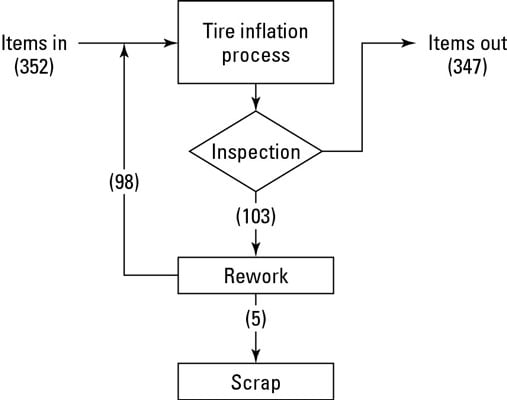# True measure of process efficiency and effectiveness

How do you measure the performance of a business process?

I would like to give you two important variables that I use to determine the efficiency and effectiveness of a business process. The first variable is called process cycle efficiency (PCE). Process cycle efficiency of an entire process is calculated by dividing the total waiting time by the total process cycle time. Let’s look an example below. Consider a three-step process to make a product x.The entire process has a cycle time of:

1. Overall cycle time = 100 +1000 + 200 + 2500 + 50 = 3850 seconds

However, there is significant waste in the process arising of items placed in queue before being worked on. This is the waiting time between processes. During this time, no value is being added to the product and items are “standing still” before being processed. Therefore, we can calculate the following variables:

1. Total waiting time = 1000 + 2500 = 3500 secs
2. Total process time = 100 + 200 + 50 = 350 secs

To calculate the efficiency of the process, we simply divide the total process time by the overall cycle time:

PCE = 350/3850 = 0.09 or 9%

Process cycle efficiency is a measure that combines both speed and value. It represents how fast a product can be produced if the system was 100% efficient, i.e. 350 secs or just under 6 mins. It also gives an indication how much value-added work was done during the production. PCE varies by application, but an average of 25 percent is considered world-class in manufacturing. (iSixSigma, n.d.)

Typical transaction and service type processes have a PCE of up to 10%. However, world class lean target would be close to 50% (Brook, 2015).

The next variable that measures the effectiveness of the process is the first-time yield (FTY) metric. Some benchmark for discrete industries is given below.

In our examples, let’s consider the process below:This time 100 units go into processing at process 1. Of which only 90 come out. This give the first time yield for process 1:

FTY_p1 = 90/100 = 0.9 (90%)

Similarly, for process 2, 90 units are input into the process 2 and 50 leave. Therefore, FTY_p2 is given by:

FTY_p2 = 50/90 = 0.56 (56%)

Finally, for process 3, 50 units enter and 40 leave, therefore the first time yield of this process is given by:

FTY_p3 =  40/50 = 0.8 (80%)

We can therefore calculate for the entire process, a first time yield of

FTY_p = 0.9 * 0.56 * 0.8 = 0.40 (40%)

So what does this tell us?

It tells us how effective the process is, if I input 100 units at process 1 then by the end of the process, for first time right yield, I will only have 40 finished units. The remaining 60 units will require rework or maybe scrapped during the process. A similar example is given below.Lean tools and techniques help increase both metrics, efficiency and effectiveness through the implementation of value stream maps, understanding the root cause for rework/defects and creating “pull” in the process.